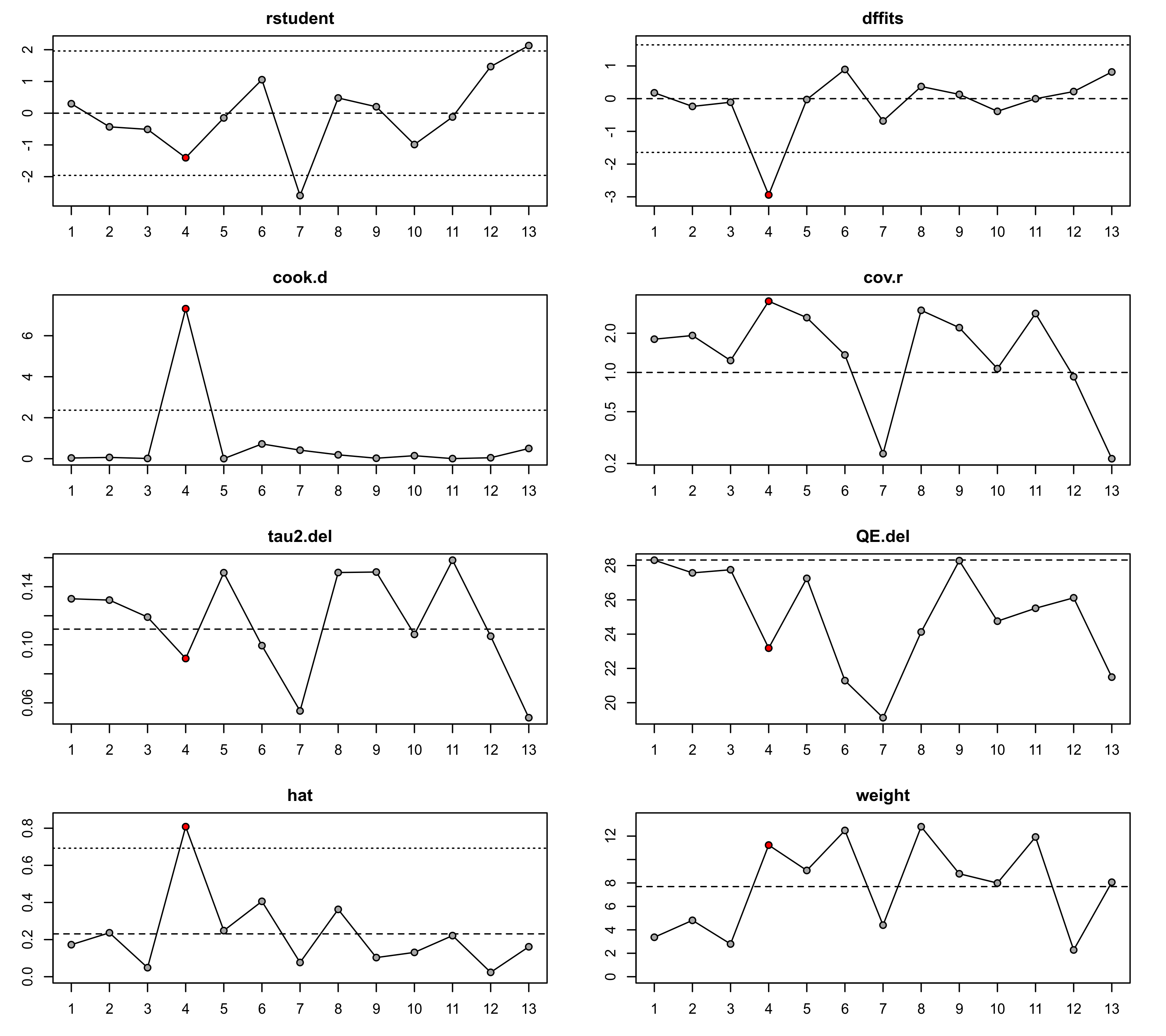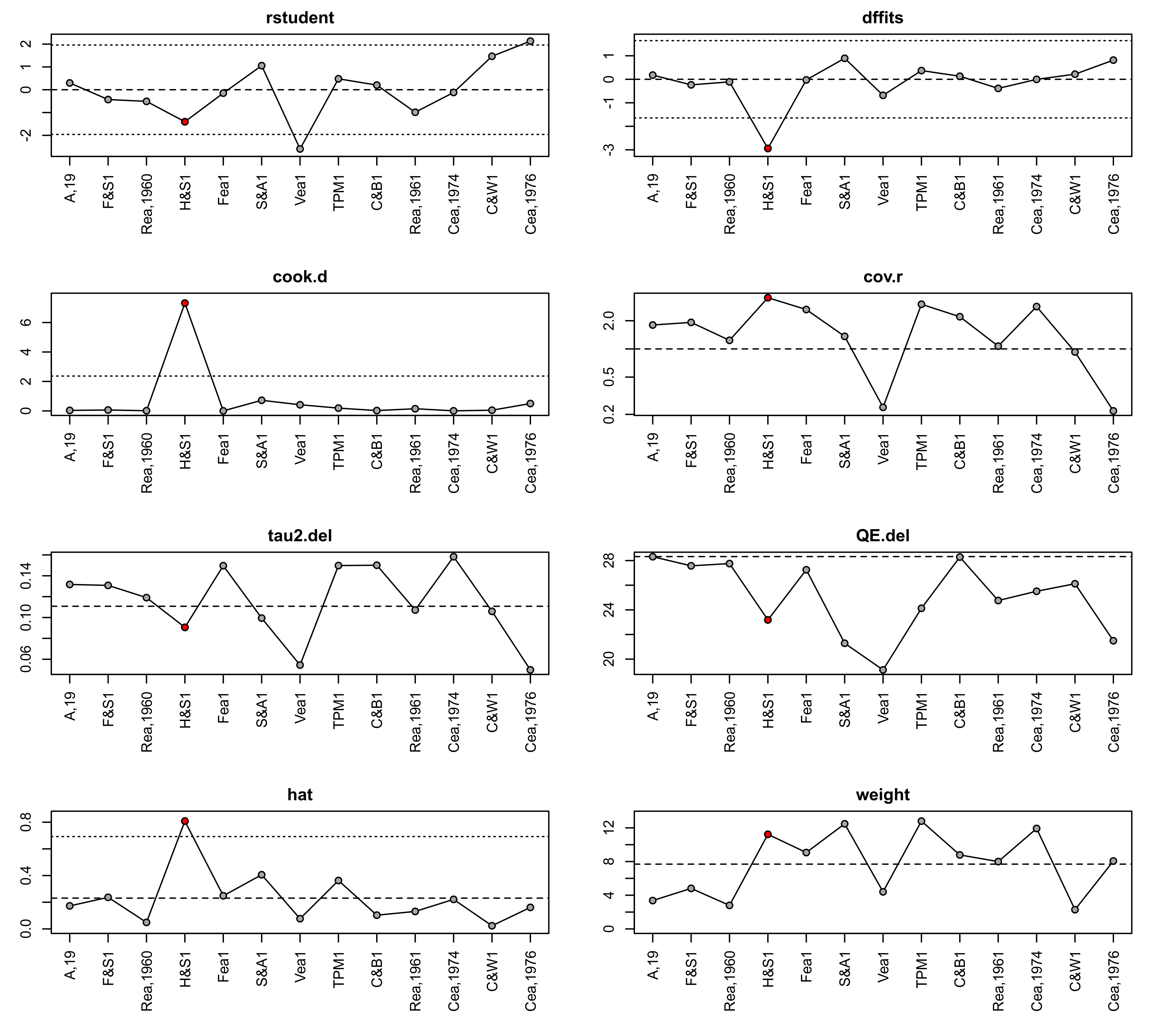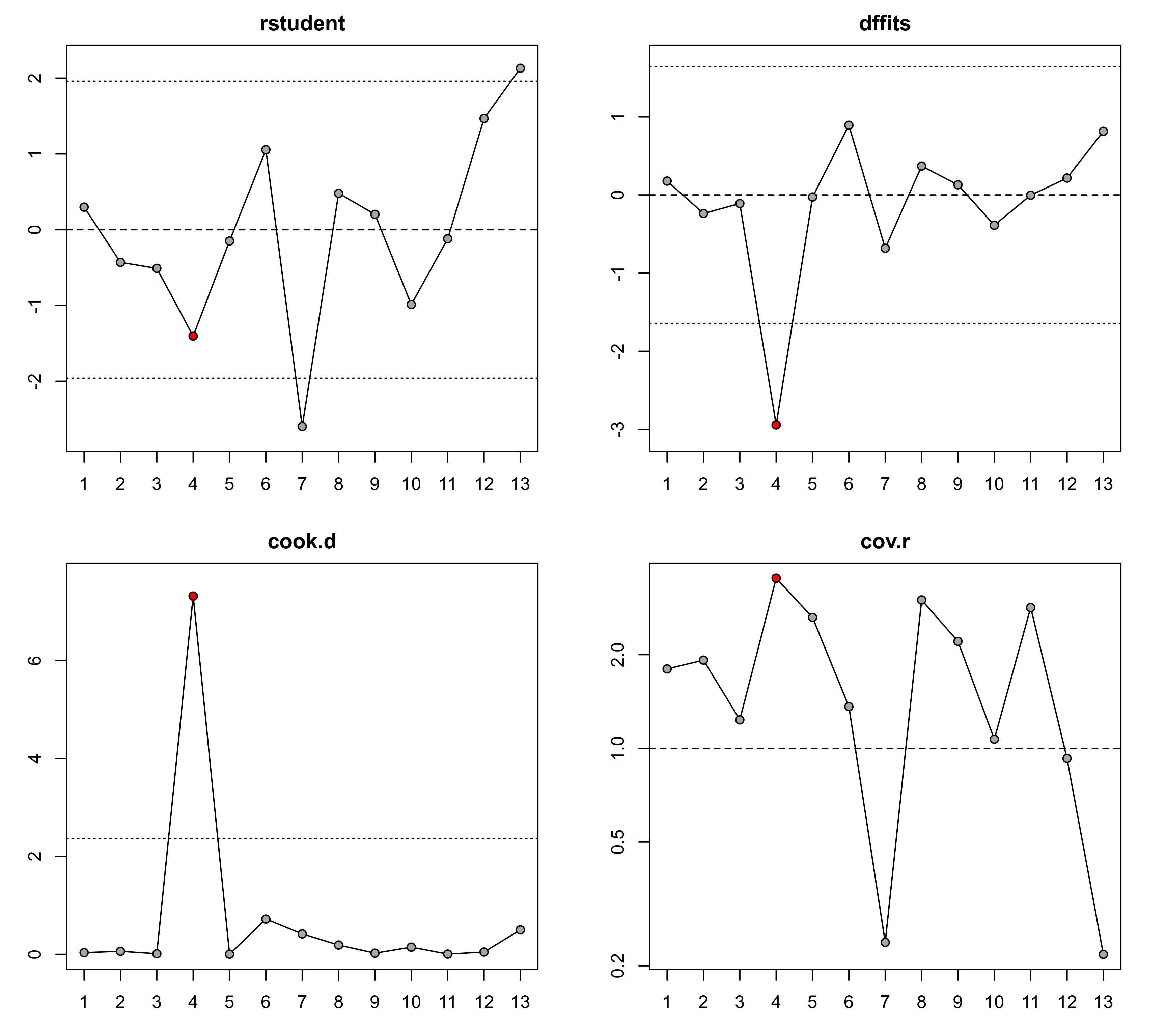Plot method for objects of class "infl.rma.uni".

# S3 method for infl.rma.uni
plot(x, plotinf=TRUE, plotdfbs=FALSE, dfbsnew=FALSE, logcov=TRUE,
layout, slab.style=1, las=0, pch=21, bg="black",
bg.infl="red", col.na="lightgray", ...)

## Arguments

x

an object of class "infl.rma.uni" obtained with influence.

plotinf

logical to specify whether the various case diagnostics should be plotted (the default is TRUE). Can also be a vector of up to 8 integers to specify which plots to draw. See ‘Details’ for the numbers corresponding to the various plots.

plotdfbs

logical to specify whether the DFBETAS values should be plotted (the default is FALSE). Can also be a vector of integers to specify for which coefficient(s) to plot the DFBETAS values.

dfbsnew

logical to specify whether a new device should be opened for plotting the DFBETAS values (the default is FALSE).

logcov

logical to specify whether the covariance ratios should be plotted on a log scale (the default is TRUE).

layout

optional vector of two numbers to specify the number of rows and columns for the layout of the figure.

slab.style

integer to indicate the style of the x-axis labels: 1 = study number, 2 = study label, 3 = abbreviated study label. Note that study labels, even when abbreviated, may be too long to fit in the margins.)

las

integer between 0 and 3 to specify the alignment of the axis labels (see par). The most useful alternative to 0 is 3, so that the x-axis labels are drawn vertical to the axis.

pch

plotting symbol to use. By default, a filled circle is used. See points for other options.

bg

color to use for filling the plotting symbol (the default is "black").

bg.infl

color to use for filling the plotting symbol when the point is considered influential (the default is "red").

col.na

color to use for lines connecting two points with NA values in between (the default is "lightgray").

...

other arguments.

## Details

When plotinf=TRUE, the function plots the (1) externally standardized residuals, (2) DFFITS values, (3) Cook's distances, (4) covariance ratios, (5) leave-one-out $$\tau^2$$ estimates, (6) leave-one-out (residual) heterogeneity test statistics, (7) hat values, and (8) weights. If plotdfbs=TRUE, the DFBETAS values are also plotted either after confirming the page change (if dfbsnew=FALSE) or on a separate device (if dfbsnew=TRUE).

A case (which is typically synonymous with study) may be considered to be ‘influential’ if at least one of the following is true:

• The absolute DFFITS value is larger than $$3 \times \sqrt{p/(k-p)}$$, where $$p$$ is the number of model coefficients and $$k$$ the number of cases.

• The lower tail area of a chi-square distribution with $$p$$ degrees of freedom cut off by the Cook's distance is larger than 50%.

• The hat value is larger than $$3 \times (p/k)$$.

• Any DFBETAS value is larger than $$1$$.

Cases which are considered influential with respect to any of these measures are indicated by the color specified for the bg.infl argument (the default is "red").

The cut-offs described above are indicated in the plot with horizontal reference lines. In addition, on the plot of the externally standardized residuals, horizontal reference lines are drawn at -1.96, 0, and 1.96. On the plot of the hat values, a horizontal reference line is drawn at $$p/k$$. Since the sum of the hat values is equal to $$p$$, the value $$p/k$$ indicates equal hat values for all $$k$$ cases. Finally, on the plot of weights, a horizontal reference line is drawn at $$100/k$$, corresponding to the value for equal weights (in %) for all $$k$$ cases. Note that all weights will automatically be equal to each other when using unweighted model fitting. Also, the hat values will be equal to the weights values (except for their scaling) in models without moderators.

The chosen cut-offs are (somewhat) arbitrary. Substantively informed judgment should always be used when examining the influence of each case on the results.

## Author

Wolfgang Viechtbauer wvb@metafor-project.org https://www.metafor-project.org

influence.rma.uni for the function to compute the various model diagnostics.

## Examples

### calculate log risk ratios and corresponding sampling variances
dat <- escalc(measure="RR", ai=tpos, bi=tneg, ci=cpos, di=cneg, data=dat.bcg)

### fit mixed-effects model with absolute latitude and publication year as moderators
res <- rma(yi, vi, mods = ~ ablat + year, data=dat)

### compute the diagnostics
inf <- influence(res)

### plot the values
plot(inf)### select which plots to show
plot(inf, plotinf=1:4)
plot(inf, plotinf=1:4, layout=c(4,1))### plot the DFBETAS values
plot(inf, plotinf=FALSE, plotdfbs=TRUE)